## RS Aggarwal Class 8 Solutions Chapter 9 Percentage Ex 9A

These Solutions are part of RS Aggarwal Solutions Class 8. Here we have given RS Aggarwal Solutions Class 8 Chapter 9 Percentage Ex 9A.

Other Exercises

Question 1.
Solution:
(i) 48% = $$\\ \frac { 48 }{ 100 }$$ = $$\\ \frac { 12 }{ 25 }$$
(ii) 220% = $$\\ \frac { 220 }{ 100 }$$ = $$\\ \frac { 11 }{ 5 }$$
(iii) 2.5% = $$\\ \frac { 2.5 }{ 100 }$$ = $$\\ \frac { 25 }{ 10X100 }$$ = $$\\ \frac { 1 }{ 40 }$$

Question 2.
Solution:
(i) 6% = $$\\ \frac { 6 }{ 100 }$$ = 0.06
(ii) 72% = $$\\ \frac { 72 }{ 100 }$$ = 0.72
(iii) 125% = $$\\ \frac { 125 }{ 100 }$$ = 1.25

Question 3.
Solution: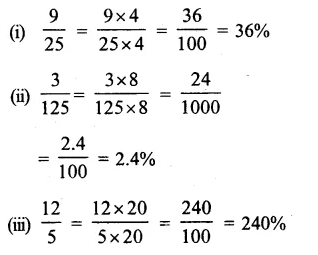Question 4.
Solution:
Ratio is 4:5 or $$\\ \frac { 4 }{ 5 }$$
$$\\ \frac { 4 }{ 5 }$$
= $$\\ \frac { 4X20 }{ 5X20 }$$
= $$\\ \frac { 80 }{ 100 }$$
= 80%

Question 5.
Solution:
125% = $$\\ \frac { 125 }{ 100 }$$
= $$\\ \frac { 5 }{ 4 }$$ (dividing by 25)
Ratio = 5:4

Question 6.
Solution: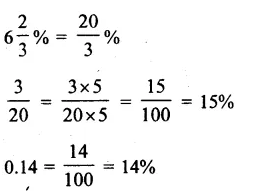We see that 15% or $$\\ \frac { 3 }{ 20 }$$ is the largest

Question 7.
Solution:
(i) Let x% of 150
= 96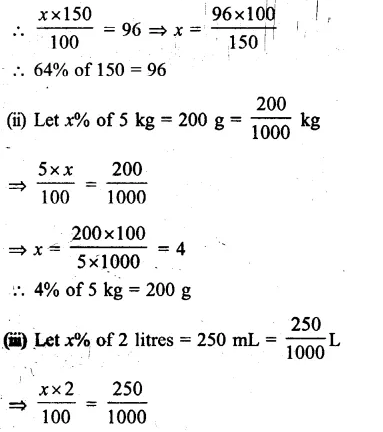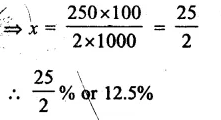Question 8.
Solution:
$$6\frac { 2 }{ 3 } %$$ of Rs 3600
= Rs $$\\ \frac { 9 }{ 2 }$$ x $$\\ \frac { 3600 }{ 100 }$$
= Rs 162

Question 9.
Solution:
16% of a number = 72
Number = $$\\ \frac { 72 }{ 16 }$$%
= $$\\ \frac { 72X100 }{ 16 }$$
= 450

Question 10.
Solution:
Let monthly income = Rs x
Savings = 18%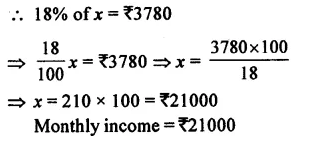Question 11.
Solution:
Let total games = x
Then game which a team wins = 73
and it is 35% of total games
35% of x = 7
=> x = $$\\ \frac { 7X100 }{ 35 }$$ = 20
Number of total games = 20

Question 12.
Solution:
Let salary = Rs x
Increment = 20%
Total salary = x + 20% of x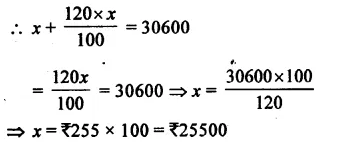Question 13.
Solution:
No. of days, Sonal attended = 204 days
and her attendance = 85% of total days
85% of total days = 204
Total number of days = $$\\ \frac { 204X100 }{ 85 }$$
= 240 days

Question 14.
Solution:
Let B’s income = Rs. 100
Then A’s income = 20% less than B’s
= 100 – 20 = Rs. 80
Difference = 100 – 80 = 20
B’s more income that A’s = $$\\ \frac { 20 }{ 80 }$$ x 100
= 25%

Question 15.
Solution:
Increase in price of petrol = 10%
Let first price = Rs. 100 p.l.
Increased price = 100 + 10 = Rs. 110
Now reduction in consumption = 110 – 100= 10
Percentage reduced consumption
= $$\\ \frac { 10X100 }{ 110 }$$
= $$\\ \frac { 100 }{ 11 }$$
= $$9\frac { 1 }{ 11 } %$$

Question 16.
Solution:
Present population of a town = 54000
Rate of increase = 8% annually
Population a year ago = $$\\ \frac { 54000X100 }{ 100+8 }$$
= $$\\ \frac { 54000X100 }{ 108 }$$
= 50000

Question 17.
Solution:
Depreciation in the value of machine = 20%
Present value = Rs. 160000
Then value of machine one year ago
= $$\\ \frac { 160000X100 }{ 100-20 }$$
= $$\\ \frac { 160000X100 }{ 80 }$$
= Rs 200000

Question 18.
Solution:
In an alloy,
Copper = 40%
Nickel = 32%
Zinc = 100 – (40% + 32%)
= 100 – 72 = 28%
Then mass of zinc in one kg of alloy
= 1 kg X 28%
= $$\\ \frac { 1000X28 }{ 100 }$$ g
= 280 gm

Question 19.
Solution:
In balance diet,
Protein = 12%
Fats = 25%
Carbohydrates = 63%
Total number of dories = 2600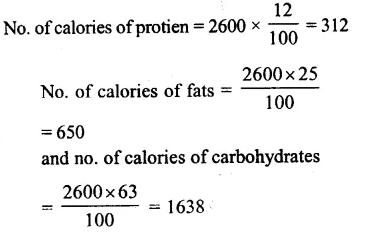Question 20.
Solution:
In gunpowder,
Nitre = 75%
Sulphur = 10%
(i) Amount of gunpowder if nitre is 9 kg
= $$\\ \frac { 100 }{ 75 }$$ x 9
= 12 kg
(ii) Amount of gunpowder if sulphur is 2.5kg 100
= $$\\ \frac { 100 }{ 10 }$$ x 2.5
= 25 kg

Question 21.
Solution:
Let C get = Rs. x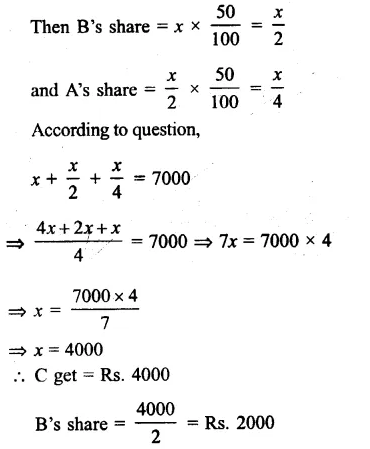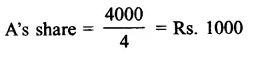Question 22.
Solution:
24-carat gold is 100% pure
22 parts out of 24 part in 22-carat goldQuestion 23.
Solution:
Let present salary = Rs. 100
Increase = 25%
Increased salary = Rs. 100 + 25
= Rs. 125
To receive the original salary, amount to be decreased = Rs. 125 – 100 = Rs. 25
∴ % decrease = $$\\ \frac { 25X100 }{ 125 }$$ = 20%

Hope given RS Aggarwal Solutions Class 8 Chapter 9 Percentage Ex 9A are helpful to complete your math homework.

If you have any doubts, please comment below. Learn Insta try to provide online math tutoring for you.